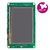## Drawing a triangle

I need to draw a triangle, i found in the stm32746g_discovery_lcd.h

#### stm32746g_discovery_lcd.h line 227

``` BSP_LCD_DrawPolygon(pPoint Points, uint16_t PointCount);
```

and i have no idea how i would set that up, i found in the stm32746g_discovery_lcd.c file where it references and the code is as follows.

#### stm32746g_discovery_lcd.c line 956

```void BSP_LCD_DrawPolygon(pPoint Points, uint16_t PointCount)
{
int16_t x = 0, y = 0;

if(PointCount < 2)
{
return;
}

BSP_LCD_DrawLine(Points->X, Points->Y, (Points+PointCount-1)->X, (Points+PointCount-1)->Y);

while(--PointCount)
{
x = Points->X;
y = Points->Y;
Points++;
BSP_LCD_DrawLine(x, y, Points->X, Points->Y);
}
}

```

I remember learning about the arrow operators and how they typically reference unions or arrays or something like that but i can't for the life of me remember exactly how to create what it needs. (and embarrassingly enough i remember this being the primary subject for nearly 3 days of classes)

Would someone please mind explaining this to me? or in the very least set up a simple 3 point triangle of some random coordinates for me to use as a reference? Thank you!

PS: BSP_LCD_FillPolygon would also work, but appears similar

#### Question relating to:The STM32F746G-DISCO discovery board (32F746GDISCOVERY) is a complete demonstration and development platform for STMicroelectronics ARM® Cortex®-M7 core-based STM32F746NGH6 microcontroller.

##### 2 years, 10 months ago.

Hi there,

you need to follow the comments above the function

#### Quote:

/**

• @brief Draws an poly-line (between many points).
• @param Points: Pointer to the points array
• @param PointCount: Number of points
• @retval None
• /

So you need an array of coordinates and when you will look into parameters of the `BSP_LCD_DrawPolygon(point Points, uint16_t PointCount);` a struct of Point must exist.

#### One struct of stm32746g_discovery_lcd.c

```typedef struct
{
int16_t X;
int16_t Y;
}Point, * pPoint;
```

So you need to add something like this, for example.

```        Point p; // tringle = 3 points = 3x struct of coordinates
p.X = 240;   p.Y = 20;
p.X = 200;   p.Y = 100;
p.X = 280;   p.Y = 100;
BSP_LCD_SetTextColor(LCD_COLOR_RED);
BSP_LCD_DrawPolygon(p, 3);
```

You can simple copy & paste it into ST example, between lines 32-33.

#### Import programDISCO-F746NG_LCD_demo

Basic example showing how to drive the LCD.

BR, Jan